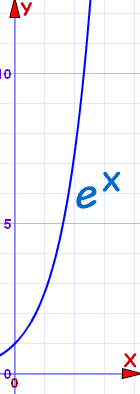# Exponential Growth and Decay

### Exponential growth can be amazing!

The idea: something always grows in relation to its current value, such as always doubling.

## Amazing TreeLet us say we have this special tree.

It grows exponentially , following this formula:

Height (in mm) = ex

e is Euler's number, about 2.718• At 1 year old it is: e1 = 2.7 mm high ... really tiny!
• At 5 years it is: e5 = 148 mm high ... as high as a cup
• At 10 years: e10 = 22 m high ... as tall as a building
• At 15 years: e15 = 3.3 km high ... 10 times the height of the Eiffel Tower
• At 20 years: e20 = 485 km high ... up into space!

No tree could ever grow that tall.
So when people say "it grows exponentially" ... just think what that means.

## Growth and Decay

But sometimes things can grow (or the opposite: decay) exponentially, at least for a while.

So we have a generally useful formula:

y(t) = a × ekt

Where y(t) = value at time "t"
a = value at the start
k = rate of growth (when >0) or decay (when <0)
t = time

### Example: 2 months ago you had 3 mice, you now have 18.Assuming the growth continues like that What is the "k" value? How many mice 2 Months from now? How many mice 1 Year from now?

y(t) = a × ekt

We know a=3 mice, t=2 months, and right now y(2)=18 mice:

18 = 3 × e2k

Now some algebra to solve for k:

Divide both sides by 3:6 = e2k
Take the natural logarithm of both sides:ln(6) = ln(e2k)
ln(ex)=x, so:ln(6) = 2k
Swap sides:2k = ln(6)
Divide by 2:k = ln(6)/2

Notes:

• The step where we used ln(ex)=x is explained at Exponents and Logarithms.
• we could calculate k ≈ 0.896, but it is best to keep it as k = ln(6)/2 until we do our final calculations.

We can now put k = ln(6)/2 into our formula from before:

y(t) = 3 e(ln(6)/2)t

Now let's calculate the population in 2 more months (at t=4 months):

y(4) = 3 e(ln(6)/2)×4 = 108

And in 1 year from now (t=14 months):

y(14) = 3 e(ln(6)/2)×14 = 839,808

That's a lot of mice! I hope you will be feeding them properly.

## Exponential Decay

Some things "decay" (get smaller) exponentially.

### Example: Atmospheric pressure (the pressure of air around you) decreases as you go higher.

It decreases about 12% for every 1000 m: an exponential decay.

The pressure at sea level is about 1013 hPa (depending on weather).• Write the formula (with its "k" value),
• Find the pressure on the roof of the Empire State Building (381 m),
• and at the top of Mount Everest (8848 m)

y(t) = a × ekt

We know

• a (the pressure at sea level) = 1013 hPa
• t is in meters (distance, not time, but the formula still works)
• y(1000) is a 12% reduction on 1013 hPa = 891.44 hPa

So:

891.44 = 1013 ek×1000

Now some algebra to solve for k:

Divide both sides by 1013:0.88 = e1000k
Take the natural logarithm of both sides:ln(0.88) = ln(e1000k)
ln(ex)=x, so:ln(0.88) = 1000k
Swap sides:1000k = ln(0.88)
Divide by 1000:k = ln(0.88)/1000

Now we know "k" we can write:

y(t) = 1013 e(ln(0.88)/1000)×t

And finally we can calculate the pressure at 381 m, and at 8848 m:

y(381) = 1013 e(ln(0.88)/1000)×381 = 965 hPa

y(8848) = 1013 e(ln(0.88)/1000)×8848 = 327 hPa

(In fact pressures at Mount Everest are around 337 hPa ... good calculations!)

## Half Life

The "half life" is how long it takes for a value to halve with exponential decay.

Commonly used with radioactive decay, but it has many other applications!

### Example: The half-life of caffeine in your body is about 6 hours. If you had 1 cup of coffee 9 hours ago how much is left in your system?y(t) = a × ekt

We know:

• a (the starting dose) = 1 cup of coffee!
• t is in hours
• at y(6) we have a 50% reduction (because 6 is the half life)

So:

0.5 = 1 cup × e6k

Now some algebra to solve for k:

Take the natural logarithm of both sides:ln(0.5) = ln(e6k)
ln(ex)=x, so:ln(0.5) = 6k
Swap sides:6k = ln(0.5)
Divide by 6:k = ln(0.5)/6

Now we can write:

y(t) = 1 e(ln(0.5)/6)×t

In 6 hours:

y(6) = 1 e(ln(0.5)/6)×6 = 0.5

Which is correct as 6 hours is the half life

And in 9 hours:

y(9) = 1 e(ln(0.5)/6)×9 = 0.35

After 9 hours the amount left in your system is about 0.35 of the original amount. Have a nice sleep :)

Have a play with the Half Life of Medicine Tool to get a good understanding of this.